### Statistics Class 10th Mathematics Tamilnadu Board Solution

##### Question 1.The range of the first 10 prime numbers 2, 3, 5, 7, 11, 13, 17, 19, 23, 29 isA. 28B. 26C. 29D. 27Answer:Range R = xmax - xminR = 29 - 2 = 27So the correct option is D.Question 2.The least value in a collection of data is 14.1. If the range of the collection is 28.4, then the greatest value of the collection isA. 42.5B. 43.5C. 42.4D. 42.1Answer:Given xmin = 14.1, R = 28.4Range R = xmax - xmin28.4 = xmax - 14.1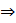xmax = 42.5So the correct option is A.Question 3.The greatest value of a collection of data is 72 and the least value is 28. Then the coefficient of range isA. 44B. 0.72C. 0.44D. 0.28Answer:Given xmax = 72, xmin = 28Coefficient of range s =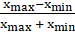=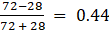So the correct option is C.Question 4.For a collection of 11 items,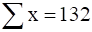, then the arithmetic mean isA. 11B. 12C. 14D. 13Answer:N = 11AM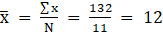So the correct option is B.Question 5.For any collection of n items,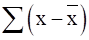=A.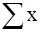B.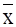C.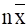D. 0Answer: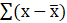can be rewritten as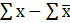We know that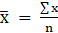so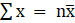Also,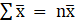because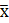is just a constant term.Substituting these in, we get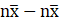= 0So the correct option is D.Question 6.For any collection of n items,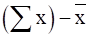=A.B.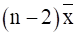C.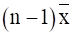D. 0Answer:We know thatsoSubstituting this in the question, we have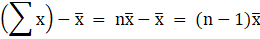So the correct option is C.Question 7.If t is the standard deviation of x, y. z, then the standard deviation of x + 5, y + 5, z + 5 isA.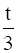B. t + 5C. tD. x y zAnswer:It is a direct consequence of the theory of standard deviation that, the same constant term added to the terms in a set of data doesn’t change the value of standard deviation.So the correct answer is t and correct option is C.Question 8.If the standard deviation of a set of data is 1.6, then the variance isA. 0.4B. 2.56C. 1.96D. 0.04Answer:Variance = SD2So Variance = 1.62 = 2.56Therefore, the correct option is B.Question 9.If the variance of a data is 12.25, then the S.D isA. 3.5B. 3C. 2.5D. 3.25Answer:Variance = SD2Or SD =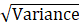SD =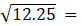3.5So the correct option is A.Question 10.Variance of the first 11 natural numbers isA.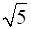B.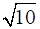C.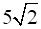D. 10Answer:Variance of first n natural numbers is given by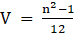V =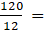10So the correct option is D.Question 11.The variance of 10, 10, 10, 10, 10 isA. 10B.C. 5D. 0Answer:Standard deviation of a constant set of data is 0.We know that Variance = SD2Variance = 0So the correct option is D.Question 12.If the variance of 14, 18, 22, 26, 30 is 32, then the variance of 28, 36,44,52,60 isA. 64B. 128C. 32√2D. 32Answer:Two data sets are given.Set A : 14, 18, 22, 26, 30 with V = 32Set B : 28, 36, 44, 52, 60 with V = ?We notice that each data entry in set B is twice the corresponding data entry in set A.SD of set B = 2 × SD of set A.We know that Variance = SD2Variance of Set B = 22 × Variance of Set A.= 4 × 32 = 128.So the correct option is B.Question 13.Standard deviation of a collection of data is 2√2. If each value is multiplied by 3, then the standard deviation of the new data isA.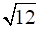B. 4√2C. 6√2D. 9√2Answer:SD of set B = n × SD of set A, where n is the data multiplierHere, n = 3.So, SD of set B = 3 × SD of set A = 3 × 2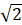= 6So, the correct option is C.Question 14.Given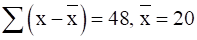and n = 12. The coefficient of variation isA. 25B. 20C. 30D. 10Answer:Insufficient data.Question 15.Mean and standard deviation of a data are 48 and 12 respectively. The coefficient of variation isA. 42B. 25C. 28D. 48Answer:CV =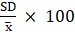CV =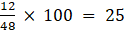So, the correct option is B.

PDF FILE TO YOUR EMAIL IMMEDIATELY PURCHASE NOTES & PAPER SOLUTION. @ Rs. 50/- each (GST extra)

HINDI ENTIRE PAPER SOLUTION

MARATHI PAPER SOLUTION

SSC MATHS I PAPER SOLUTION

SSC MATHS II PAPER SOLUTION

SSC SCIENCE I PAPER SOLUTION

SSC SCIENCE II PAPER SOLUTION

SSC ENGLISH PAPER SOLUTION

SSC & HSC ENGLISH WRITING SKILL

HSC ACCOUNTS NOTES

HSC OCM NOTES

HSC ECONOMICS NOTES

HSC SECRETARIAL PRACTICE NOTES

2019 Board Paper Solution

HSC ENGLISH SET A 2019 21st February, 2019

HSC ENGLISH SET B 2019 21st February, 2019

HSC ENGLISH SET C 2019 21st February, 2019

HSC ENGLISH SET D 2019 21st February, 2019

SECRETARIAL PRACTICE (S.P) 2019 25th February, 2019

HSC XII PHYSICS 2019 25th February, 2019

CHEMISTRY XII HSC SOLUTION 27th, February, 2019

OCM PAPER SOLUTION 2019 27th, February, 2019

HSC MATHS PAPER SOLUTION COMMERCE, 2nd March, 2019

HSC MATHS PAPER SOLUTION SCIENCE 2nd, March, 2019

SSC ENGLISH STD 10 5TH MARCH, 2019.

HSC XII ACCOUNTS 2019 6th March, 2019

HSC XII BIOLOGY 2019 6TH March, 2019

HSC XII ECONOMICS 9Th March 2019

SSC Maths I March 2019 Solution 10th Standard11th, March, 2019

SSC MATHS II MARCH 2019 SOLUTION 10TH STD.13th March, 2019

SSC SCIENCE I MARCH 2019 SOLUTION 10TH STD. 15th March, 2019.

SSC SCIENCE II MARCH 2019 SOLUTION 10TH STD. 18th March, 2019.

SSC SOCIAL SCIENCE I MARCH 2019 SOLUTION20th March, 2019

SSC SOCIAL SCIENCE II MARCH 2019 SOLUTION, 22nd March, 2019

XII CBSE - BOARD - MARCH - 2019 ENGLISH - QP + SOLUTIONS, 2nd March, 2019

HSC Maharashtra Board Papers 2020

(Std 12th English Medium)

HSC ECONOMICS MARCH 2020

HSC OCM MARCH 2020

HSC ACCOUNTS MARCH 2020

HSC S.P. MARCH 2020

HSC ENGLISH MARCH 2020

HSC HINDI MARCH 2020

HSC MARATHI MARCH 2020

HSC MATHS MARCH 2020

SSC Maharashtra Board Papers 2020

(Std 10th English Medium)

English MARCH 2020

HindI MARCH 2020

Hindi (Composite) MARCH 2020

Marathi MARCH 2020

Mathematics (Paper 1) MARCH 2020

Mathematics (Paper 2) MARCH 2020

Sanskrit MARCH 2020

Sanskrit (Composite) MARCH 2020

Science (Paper 1) MARCH 2020

Science (Paper 2)

MUST REMEMBER THINGS on the day of Exam

Are you prepared? for English Grammar in Board Exam.

Paper Presentation In Board Exam

How to Score Good Marks in SSC Board Exams

Tips To Score More Than 90% Marks In 12th Board Exam

How to write English exams?

How to prepare for board exam when less time is left

How to memorise what you learn for board exam

No. 1 Simple Hack, you can try out, in preparing for Board Exam

How to Study for CBSE Class 10 Board Exams Subject Wise Tips?

JEE Main 2020 Registration Process – Exam Pattern & Important Dates

NEET UG 2020 Registration Process Exam Pattern & Important Dates

How can One Prepare for two Competitive Exams at the same time?

8 Proven Tips to Handle Anxiety before Exams!